## Rotation

Xara calculates and generates the tween frames automatically by working out how to transform the shapes from one key frame to the next. But sometimes it's not possible to know exactly what type of rotation is required.

 Take this example. Suppose you tween from the first clock face to the second: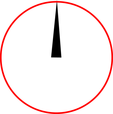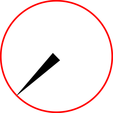You might expect it to rotate clockwise, but of course it could go counter clockwise: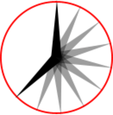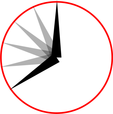In fact there are other ways it could rotate. The above examples assume the hand rotates around one end, but you could tween from one state into the other by doing a straight line rotation of the center of the object, which would result in this: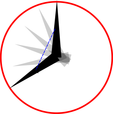If you look closely, the hand rotates around its center rather than one end, and the center point moves in the straight line from one key frame to the next. By default, Xara rotates objects this way.

### The Rotate command

To force the rotation to be around any other point you can use a special keyword "rotate" appended to the front of the object name. Or you can use "rotate +" to force the rotation to go clockwise or "rotate -" to make it go counter clockwise.

To take the simple two keyframes animation example shown on the previous page, where the hour hand needs to move from 12 o'clock to 8 o'clock. If you name the hand object "rotate + hand", you'll get an animation showing the clock's hand moving clockwise. If you name it "rotate - hand", then the hand will rotate counter clockwise.

If there is no rotate command, then the tween takes the shortest direct path between the object on one keyframe to the next, rotating around its center. With a "rotate" command on the object name, it calculates the actual rotation point, which in the above example is the "large end" of the hand.

Note: It's not possible to rotate an object by a full 360° between keyframes if the rotation point is not the center. For example, to do a full rotation of the clock hands in the above example, you would need to create an intermediate keyframe.